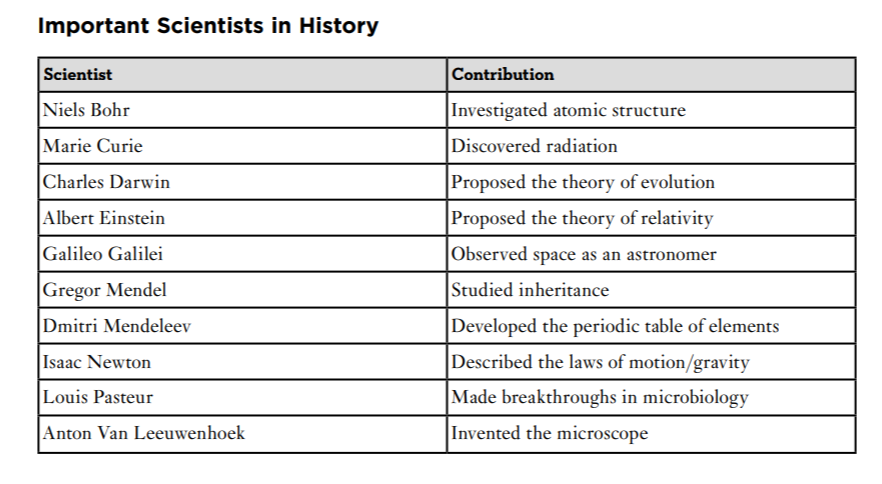Scan QR code or get instant email to install app

Question:

# Isaac Newton (1642–1727) was a physicist, mathematician, astronomer, philosopher, chemist, and theologian. One of the most important contributions Newton made to science is known as Newton’s three laws of motion. The first law states that every object in motion stays in motion. The second law states that the force of an object equals its mass times its acceleration. The third law states that for every action there is an equal and opposite reaction. Which of the following would be the BEST title for this information?A “Isaac Newton’s Three Laws of Motion”
explanation

“Isaac Newton’s Three Laws of Motion” summarizes what the article is about. “The History of Science” is much too broad; a single article cannot include the entire history of science. “Physics Explained” is not what the article is about, even though Newton was a physicist. “The Life and Times of Isaac Newton” is partially correct, but we only read about one small part of Newton’s life.